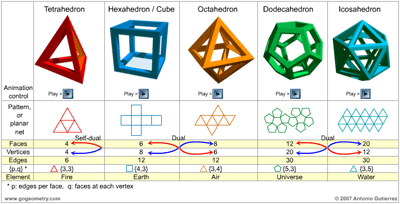#Platonic Solids, Theaetetus's Theorem, Euler's Polyhedron Theorem, HTML5 Animation for iPad

Instructions: To control the animation of each platonic solid, press the STOP/PLAY button.

Theaetetus' Theorem (ca. 417 B.C. – 369 B.C.) There are precisely five regular convex polyhedra or Platonic solid.

A platonic solid is a polyhedron all of whose faces are congruent regular polygons, and where the same number of faces meet at every vertex. A polyhedron is a solid figure bounded by plane polygons or faces.

The Greek philosopher Plato described the solids in detail in his book "Timaeus" and assigned the items to the Platonic conception of the world, hence today they are well-known under the name "Platonic Solids."

Euler's polyhedron theorem: F + V = E + 2, where F, V, E are the number of faces, vertices, and edges in the polyhedron.